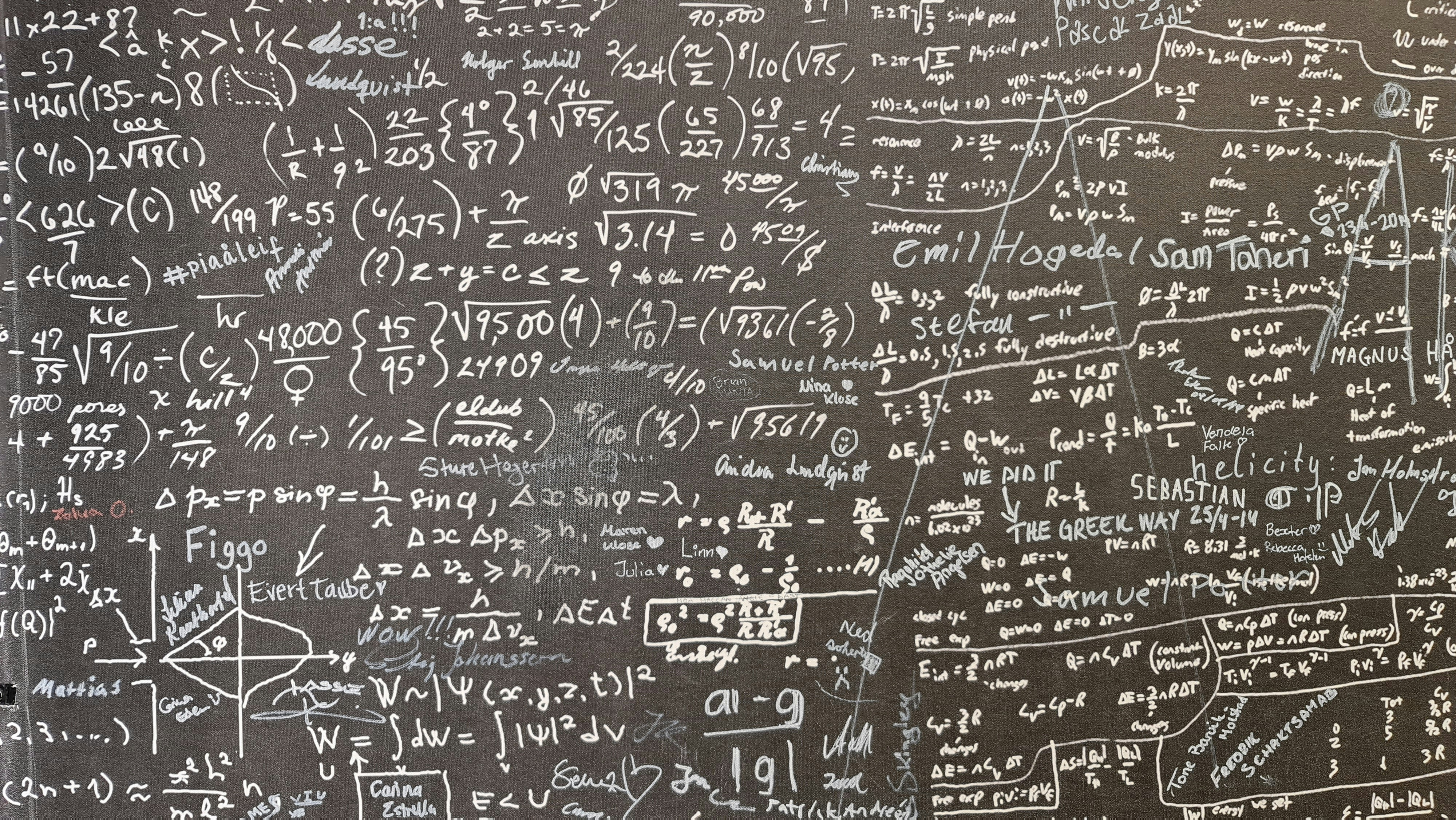# Lagrange interpolation

Date
May 26, 2023
Tags
Maths
I remember I learnt about this in my secondary school applied mathematics classes. Of course, at that time I didn’t really find that useful in real life and I almost have forgotten this. These days, I am studying zero knowledge proof and one key topic is about polynomial interpolation. That reminds me about Lagrange interpolation again. I would like to write about this interpolation and hopefully at the same time, helps me to revise the key concept behind this.
So Lagrange interpolation is a mathematical method used to find a polynomial function that goes through a set of given points. The polynomial function is used to approximate the behaviour of a given data set. It is also known as Lagrange polynomial interpolation or Lagrange's method.
Imagine a scenario that you have multiple in the coordinate plane. These and values can be time and its corresponding temperature within a day. So your task is to link up these points in the coordinate plane using a polynomial. With this polynomial, you can approximately evaluate the corresponding temperature with any give time within a day.
So say we have three points in the coordinate plane
 ㅤ 2 7 1 5 3 4
The mission is to create a polynomial that passes through all these three points. Meaning , and
Lagrange interpolation helps to come up with that polynomial by breaking down the final polynomial into three polynomials , and . So that for , it passes through the first point, but for the other values, . Similar applies to and . And later on if we add these three polynomials together, the resulting will just pass through all these three points.
The polynomial will look like this
is designed like this because if we look at the numerator, it ensures the whole polynomial will be evaluated to 0 if we are at and . For , the denominator is there to normalize the result to 1, and then we multiply it by , which is 7 in this case. That makes sure , which means this polynomial passes through the first point provided in the above table.
Applying the same concept, we can quickly come up with and . And in the general form, the Lagrange interpolation looks like this.
Where,
• and are the given data points
• is the number of data points
• is the polynomial function that passes through all the given data points

And this is how the function looks in graph
And click here if you are interested to see the code for generating the graph
import numpy as np
from matplotlib import pyplot as plt

x = np.linspace(0, 5, 100)
y1 = 7 * (x-1) * (x-3) / -1
y2 = 5 * (x-2) * (x-3) / 2
y3 = 4 * (x-2) * (x-1) / 2
y = y1 + y2 + y3

plt.plot(x, y, '-')
plt.grid(True)

plt.plot(2, 7, 'x')
plt.plot(1, 5, 'x')
plt.plot(3, 4, 'x')

plt.xlabel('x')
plt.ylabel('y')
plt.title("Lagrange Interpolation")
plt.show()
Being able to convert points into polynomial can be very useful in different fields. Apart from allowing the computer to plot a smooth and continuous line passing through these points, we can also ‘encode’ information inside a polynomial. It turns out to be one of the key tools used in cryptography, e.g. zero knowledge proof.